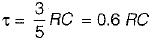Courses

# First Order RL & RC Circuits - 2

## 15 Questions MCQ Test Topicwise Question Bank for Electrical Engineering | First Order RL & RC Circuits - 2

Description
This mock test of First Order RL & RC Circuits - 2 for Electrical Engineering (EE) helps you for every Electrical Engineering (EE) entrance exam. This contains 15 Multiple Choice Questions for Electrical Engineering (EE) First Order RL & RC Circuits - 2 (mcq) to study with solutions a complete question bank. The solved questions answers in this First Order RL & RC Circuits - 2 quiz give you a good mix of easy questions and tough questions. Electrical Engineering (EE) students definitely take this First Order RL & RC Circuits - 2 exercise for a better result in the exam. You can find other First Order RL & RC Circuits - 2 extra questions, long questions & short questions for Electrical Engineering (EE) on EduRev as well by searching above.
QUESTION: 1

Solution:
QUESTION: 2

### The Laplace transform of the derivative of the signal f(t) = e-t u(t) is

Solution:

The derivative of function f(t) is
f'(t) = e-tδ (t) + u (t) (-e-t)
= -e-t u(t) + e-tδ(t)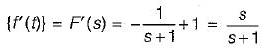QUESTION: 3

### For a series R - C circuit excited by a dc voltage of 20 V, and with time-constant τ seconds, the voltage across the capacitor at time t = τ is given by

Solution:

Vc(t) = V(1 - e-t/τ) volts
V = 20 volt (given)
At t = τ, Vc|t = τ = 20 (1 - e-1) volts

QUESTION: 4

The Laplace-transform equivalent of a given network will have 7/5 F capacitor replaced by 5

Solution: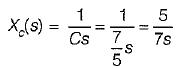QUESTION: 5

An RC circuit has a capacitor C = 2 μF in series with a resistance R = 1 MΩ The time of 6 secs will be equal to

Solution:

Time constant of series RC circuit is
τ = RC = 1 x 106 x 2 x 10-6
= 2 secs
Hence, time of 6 seconds will be equal to 3τ = 3 times of time constant.

QUESTION: 6

The Laplace transform of the gate function shown below is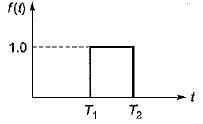Solution:

From the given waveform, we have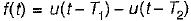∴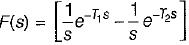QUESTION: 7

Assuming initial condition to be zero, the current i(t) in the circuit shown below is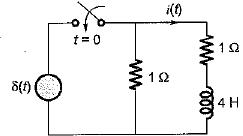Solution:

The given circuit in Laplace domain is shown below.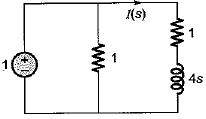Here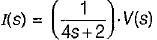(Using current divider theorem)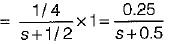or, i(t) = 0.25 e-0-5t u(t)

QUESTION: 8

A 10 volts step voltage is applied across a RC series circuit at t = 0 having R = 100 Ω, and C = 100 μF.
The values of i(t) and di(t)/dt at t = 0 are respectively given by

Solution: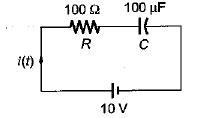At t = 0, capacitor acts as short-circuit.
So,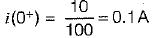Time constant of given circuit is
τ = RC = 100 x 100 x 10-6
= 10-2 sec = 0.01 sec
With zero initial condition, current in the series RC circuit is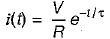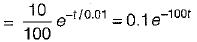Hence,
di/dt = -10 e-100t
i(0+) = 0.1 A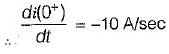QUESTION: 9

If the input voltage V1(t) = 10 e-2t V then, the output voltage V2(t) for the circuit shown below is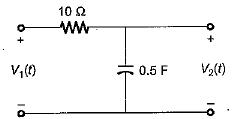Solution:

Given circuit is a low-pass filter having transfer function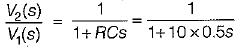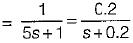When, V1(t) = 10e-2t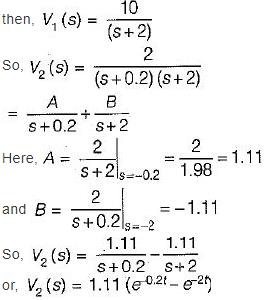QUESTION: 10

In the circuit shown below, the switch 'S' is opened at t = 0. Prior to that, switch was closed.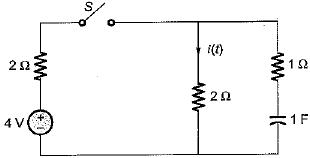The current i(t) is at t = 0+ is

Solution:

For t = 0-, switch Sis closed. Hence, in steady state capacitor will act as open circuit.
Thus, V0(0-) = VC(0+) = 2 volts
The circuit at t = 0+ is shown below where,
cananitor ants as a constant voltage source.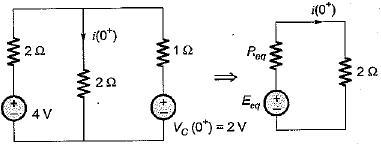Using source transformation,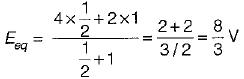andHence,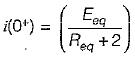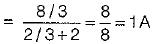QUESTION: 11

A rectangular pulse of duration T and magnitude A has the Laplace transform

Solution: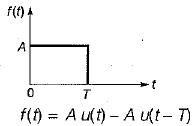∴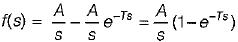QUESTION: 12

The time constant of the circuit shown below is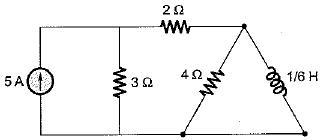Solution:

Reg across inductor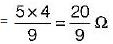(5 A C.S open circuited)
∴ Time constant of RL circuit is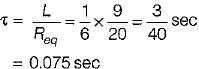QUESTION: 13

Assertion (A): Laplace transform is preferred for solving networks involving higher order differential equations.
Reason (R): The classical method for solving differential equations of higher order is quite cumbersome.

Solution:
QUESTION: 14

Assertion (A): In a purely capacitive circuit, the current wave is more distorted than the voltage wave.
Reason (R): The harmonics in the current wave are increased in proportion to their frequency numbers.

Solution:
QUESTION: 15

The time constant of the circuit shown below is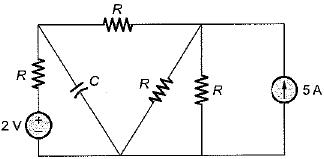Solution:

For finding time constant of the circuit 5 A current source is open circuited and 2 V voltage source is short circuited.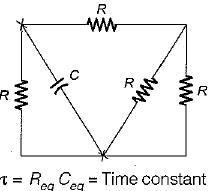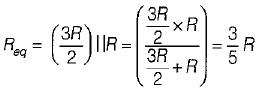Ceq = C
∴